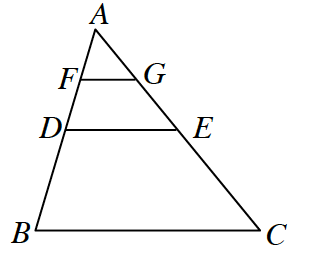### Home > CCG > Chapter 12 > Lesson 12.1.4 > Problem12-53

12-53.In the triangle at right, $\overline{DE}$ is a midsegment of $\Delta ABC$ and $\overline{FG}$ is a midsegment of$\Delta ADE$.

1. If $DE = 7$ cm, find $BC$ and $FG$.

$\Delta ADE \sim \Delta ABC$

Since $D$ is the midpoint of line $AB, 2 \cdot DB = AB$.

$FG = 3.5$ cm
$BC = 14$ cm

2. If the area of $\Delta AFG$ is $3\text{ cm}^2$, what is the area of $DECB$?

The area of a triangle is $\frac{1}{2} (b)(h)$, if the base is $3.5$ cm, and the area is $3$ cm, what is the height?

Find the areas of $\Delta ABC$ and $\Delta ADE$. Find the difference between the two triangles to find the area of $DECB$.